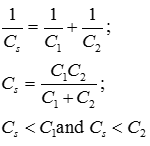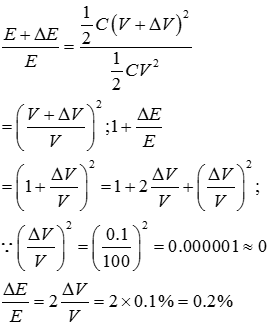# Topic: Electrostatic Potential and Capacitance (Test 1)

Topic: Electrostatic Potential and Capacitance
Q.1
Work done by a uniform electric field E in moving a charge q a distance d from a to b is
A. qEd
B. qE/d
C. q/(Ed)
D. qE/d2
Answer : Option A
Explaination / Solution:

Force and the work done
Workspace
Report
Q.2
If a positive charge moves in the direction of the electric field
A. Field does positive work on charge,potential energy increases
B. Field does positive work on charge,potential energy decreases
C. Field does negative work on charge,potential energy increases
D. Field does negative work on charge,potential energy decreases
Answer : Option B
Explaination / Solution:

A positive charge experiences a force in the direction of the field. If it moves in the direction of the field, positive work is done by the electric field on the charge. Potential energy of the charge increases only when an external source does work on the charge against the direction of the field- eg: when the charge is moved against the direction of the field

Workspace
Report
Q.3
For a one dimensional electric field the correct relation of and potential V is
A. dV/dx
B. -dV/dx
C. d2Vdx2
D. d2Vdx2
Answer : Option B
Explaination / Solution:

Electric field is defined as the negative gradient of the potential. The potential decreases in the direction of the electric field.
Workspace
Report
Q.4
Which of the following is true about equipotential lines
A. Electric field lines are tangential to equipotential lines
B. Electric field lines are perpendicular to equipotential lines
C. Electric field lines are opposite to equipotential lines
D. Electric field lines are parallel to equipotential lines
Answer : Option B
Explaination / Solution:

The potential at all points on an equipotential surface is constant and work done in moving a charge on an equipotential surface is zero. Electric field lines show the direction of the electric field at the point. If the electric field lines were tangential, parallel or opposite to the equipotential surface, a tangential field will exist on the surface and work done in moving a charge on the surface is not zero. Therefore electric field lines are always perpendicular to the equipotential surface.

Workspace
Report
Q.5
when two capacitors are put in series, the equivalent capacitance is
A. the reciprocal of the capacitances
B. the sum of the capacitances
C. smaller than both capacitances
D. the product of the capacitances
Answer : Option C
Explaination / Solution:

When two capacitors C1 and C2 are connected in series, the reciprocal of the equivalent capacitance in series is equal to the sum of the reciprocals of the two individual capacitances.It has value lesser than the least value of capacitance.

Workspace
Report
Q.6
If the dielectric constant and dielectric strength be denoted by K and X respectively, then a material suitable for use as a dielectric in a capacitor must have
A. high K and low X
B. low K and low X.
C. low K and high X
D. high K and high X
Answer : Option D
Explaination / Solution:

The capacitance of a capacitor increases with increase in the value of dielectric constant. =K C. Dielectric strength is the maximum electric field the material can withstand without breaking down. A high value of X and a high value of K is preferable for use in capacitors.

Workspace
Report
Q.7
The amount of energy a capacitor can store is called its
A. Inductance
B. Capacitance
C. resistance
D. reactance
Answer : Option B
Explaination / Solution:

Energy stored in a capacitor
Workspace
Report
Q.8
In series combination of capacitors, potential drops across the individual capacitors are equal to
A. inverse ratio of capacitors
B. inverse ratio of charges stored.
C. direct ratio of capacitors
D. direct ratio of charges stored
Answer : Option A
Explaination / Solution:

When capacitors are connected in series, they have equal charge but the potential difference across them is given by $\text{and so on}$.Therefore
Workspace
Report
Q.9
Dielectric polarization is the phenomenon
A. In which atomic dipoles are aligned opposite to the direction of electric
B. In which atomic dipoles are aligned in the direction of electric field and
C. in which atomic dipoles are aligned in the direction of electric field and
D. In which atomic dipoles are aligned opposite to the direction of the electric
Answer : Option C
Explaination / Solution:

Atomic dipoles in a dielectric experience a torque when placed in an external electric field. As a result of this torque, they align themselves in the direction of the electric field, since thi is the position of least potential energy. This phenomenon in which the atomic dipoles align themselves in the diorection of the electric field is called dielectric polarization.

Workspace
Report
Q.10
If the potential difference between the plates of a capacitor is increased by 0.1%, the energy stored in the capacitor increases by very nearly
A. 0.1%
B. 0.144%
C. 0.20%
D. 0.11%
Answer : Option C
Explaination / Solution:

The initial energy stored in the capacitor at potential difference V is When the p.d is increased by ,the energy stored is Solving,Workspace
Report